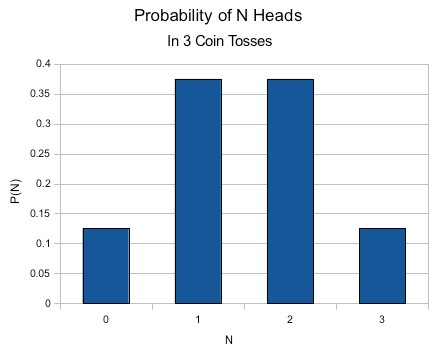# How might you construct a discrete probability distribution? Provide an example.

Suppose we want something simple, like the number of heads in 3 tosses of a coin. We list the outcomes and count those of interest. For our coin toss example, know the choices are
t t t  -  0 heads
t t h  -  1 head
t h t  -  1 head
t h h  -  2 heads
h t t  -  1 head
h t h  -  2 heads
h h t  -  2 heads
h h h  -  3 heads

Because it is easier here, we will create the distribution "sideways". Down the side, we will list the number of heads. Across we will show the probability as a bar indicating the number of instances. At the end of the bar, we will show the probability of that number of heads in 3 tosses.

N | P(N heads in 3 tosses)
0 | x  (1/8)
1 | x x x  (3/8)
2 | x x x  (3/8)
3 | x  (1/8)

With a little more effort, one can use the graphing capabilities of spreadsheet software to create a more conventional distribution graph.thanked the writer.
Oddman commented
If your question is about creating a set of discrete data with a particular distribution and other particular characteristics (autocorrelation, spectral density, and so on), that is another kettle of fish. I'm sure entire books have been written on those subjects.